## Two Populations; Independent Samples -- Variance

### Preliminaries

This topic is quite different from the others presented in this section. First, we will be looking at the variance as a parameter of a population or as a statistic of a sample. Up to this point we have generally been dealing with the standard deviation rather than the variance. Remember that the variance is just the square of the standard deviation. For populations σ is the standard deviation and σ2 is the variance. For samples s is the standard deviation and s2 is the variance. Second, we will be looking at two populations, and therefore at two independent random samples, but the underlying populations must be normal (or at least really close to normal). Third, we will be looking at the ratio of values, as in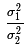or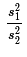. Fourth, we will be using a new distribution, the F-distribution, which, if we were using tables of values, would be dramatically more complex than any distribution that we have seen to this point. However, since we are using R there is just a slight up-tick in the complexity of using this new distribution.

### The F-distribution

This section is meant to be enlightening, but not a thorough treatment of the F-distribution. In earlier distributions, the binomial, the normal, the Student's t, and the χ² we have actually gone back to looking at values in tables. You may have noticed the following progression to which we now add the F distribution.
• normal distribution: We have one standard table which we can read forward or backward. The normal distribution is symmetric. z-values for the normal distribution can be positive or negative, with the vast majority of the standard normal distribution lying between the z-values -3 to 3. In R, rather than read a table, we use the functions pnorm and qnorm.

The function `pnorm(z)` gives us the area under the curve to the left of the z-score `z`, i.e., the probability of getting a value less than `z` in the standard normal distribution.

The function `pnorm(z,lower.tail=FALSE)` gives us the area under the curve to the right of the z-score `z`, i.e., the probability of getting a value greater than `z` in the standard normal distribution.

The function `qnorm(p)` gives us the z-score in a standard normal distribution that has the area under the curve to the left of z being equal to `p`, i.e., that has a probability of `p` of being less than z.

The function `qnorm(p,lower.tail=FALSE)` gives us the z-score in a standard normal distribution that has the area under the curve to the right of z being equal to `p`, i.e., that has a probability of `p` of being greater than z.

• Student's t distribution: We could have a different standard distribution table for each different number of degrees of freedom and we could read these tables forward and backward. However, the practice is to have a single table that just holds critical values for just a few common percentages. t-scores for the Student's t distribution can be positive or negative and the spread of the scores changes with the change in the degrees of freedom. For lower degrees of freedom the Student's t is significantly flatter than was the normal distribution. However for large degrees of freedom the Student's t approaches the normal distribution. The Student's t distribution is symmetric. In R, rather than read a table, we use the functions pt and qt.

The function `pt(t,df)` gives us the area under the curve, for `df` degrees of freedom, to the left of the t-score `t`, i.e., the probability of getting a value less than `t` in the student's-t distribution for `df` degrees of freedom.

The function `pt(t,df,lower.tail=FALSE)` gives us the area under the curve, for `df` degrees of freedom, to the right of the t-score `t`, i.e., the probability of getting a value greater than `t` in the student's-t distribution for `df` degrees of freedom.

The function `qt(p,df)` gives us the t-score in a student's-t distribution with `df` degrees of freedom that has the area under the curve to the left of t being equal to `p`, i.e., that has a probability of `p` of being less than t for `df` degrees of freedom.

The function `qt(p,df,lower.tail=FALSE)` gives us the t-score in a student's-t distribution with `df` degrees of freedom that has the area under the curve to the right of t being equal to `p`, i.e., that has a probability of `p` of being greater than t for `df` degrees of freedom.

• χ² distribution: We could have a different standard distribution table for each different number of degrees of freedom and we could read these tables forward and backward. However, the practice is to have a single table that just holds critical values for just a few common percentages. The χ² distribution is not symmetric. The x-values of the χ² distribution must be non-negative. The distribution changes shape with different degrees of freedom. In an earlier page we saw examples of graphs of various χ² distributions. In R, rather than read a table, we use the functions pchisq and qchisq.

The function `pchisq(x,df)` gives us the area under the curve, for `df` degrees of freedom, to the left of the χ²-score `x`, i.e., the probability of getting a value less than `x` in the χ² distribution for `df` degrees of freedom.

The function `pchisq(x,df,lower.tail=FALSE)` gives us the area under the curve, for `df` degrees of freedom, to the right of the χ²-score `x`, i.e., the probability of getting a value greater than `x` in the χ² distribution for `df` degrees of freedom.

The function `qchisq(p,df)` gives us the x-score in a χ² distribution with `df` degrees of freedom that has the area under the curve to the left of x being equal to `p`, i.e., that has a probability of `p` of being less than x for `df` degrees of freedom.

The function `qchisq(p,df,lower.tail=FALSE)` gives us the x-score in a χ² distribution with `df` degrees of freedom that has the area under the curve to the right of x being equal to `p`, i.e., that has a probability of `p` of being greater than x for `df` degrees of freedom.

• The F distribution: This is a non-symmetric distribution with two different degrees of freedom. Thus, we could generate a table for each different pair of degrees of freedom and we could read these tables forwards and backwards. This is even worse than you might imagine in that the pair of degrees of freedom 4,7 is different from the pair 7,4. Here is a link to a page that allows you to generate a specific table for a specific pair of degrees of freedom. To print these we would need a different table for each pair of values.

Alternatively, we could look at critical values of the F-distribution, and then we could have a different table for each possible value of the degrees of freedom of the numerator. Here is a link to a page that allows you to generate a specific table for a given numerator degrees of freedom. To print these we would need a different table for each possible numerator value.

However, the practice is to have either three or four tables of critical values, one for each of three or four special percentages, or to have just one table of critical values where each row-column intersection holds three or four values, one for each of three or four special percentages. Here is a link to a page that allows you to generate a specific table for a given area in the right tail. We would need a different table for each desired area in the right tail. If all of this seem complicated then you are correct and you can see the beauty of using software such as R to find values for the F distribution..

The x-values for the F distribution must be non-negative. The shape of F distribution changes for different pairs of degrees of freedom. Images of this are shown below.
Not that we will really use the images of the F distribution, but it is worth seeing some of them so that we get a sense of how the distribution depends upon the degrees of freedom and so that we can see the changing range of the x-values. Figure 1 shows the distribution for the degrees of freedom pair 60,60.

Figure 1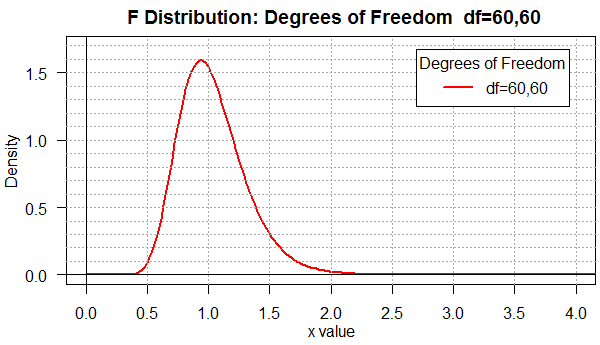Among the many things to notice in Figure 1 are the facts that most of the area is between x-values 0.4 and 2.0 and that the distribution is not symmetric (note that most of the rise occurs between 0.5 and 1 while the fall is spread out, for the most part, between 1 and 2.

When we move to degree of freedom pairs that are not "balanced" as in the pair 60,10 (shown in Figure 2) or the pair 10,60 (shown in Figure 3), we see a real change in the shape of the curve.
 Figure 2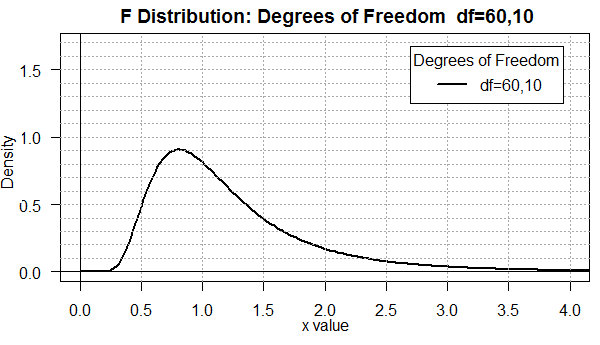Figure 3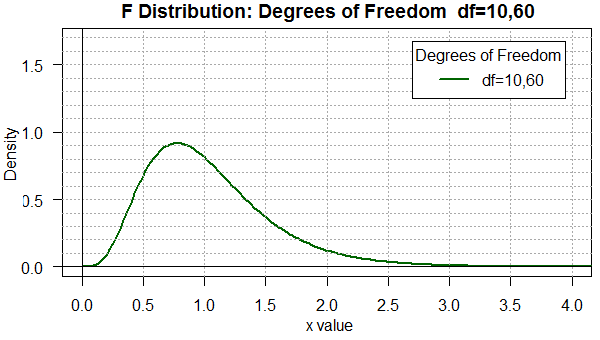You should be able to see that there is a difference in the two distributions even though the only change is to reverse the degrees of freedom. Furthermore, in both cases, the area under the curve is more spread out than it was in Figure 1. Now we find appreciable area for x-values below 0.5 and above 2.0.

Figure 4 uses yet another degree of freedom pair. This one, 8,40, is similar to the 10,60 we just saw in Figure 3, but, again, the graph is noticeably different.

Figure 4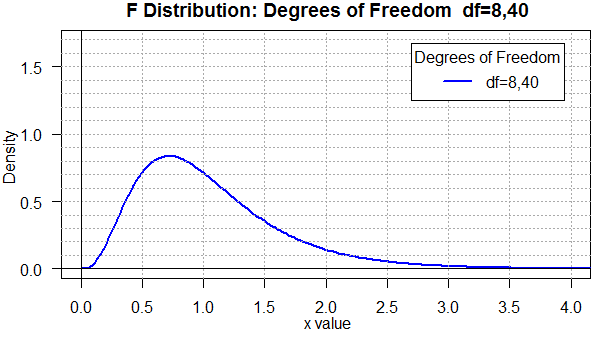To help compare all four of the previous graphs, Figure 5 places them all on one plot.

Figure 5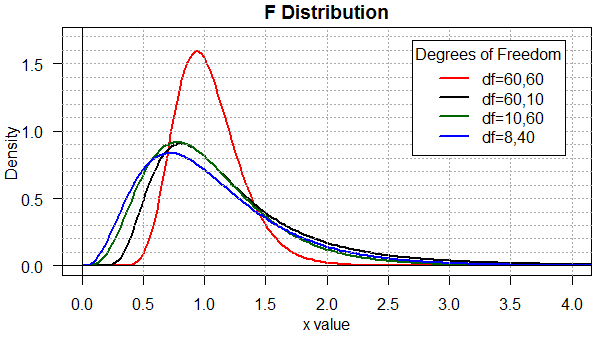Finally, toward the end of this web page you will find the R commands used to produce these five graphs. It is worth making a copy of those commands and altering them appropriately to investigate other degree of freedom pairs.

### The ratio of variances

Up to this point when we want to talk about two parameters or statistics being equal we looked at their difference and said that if they are the same then their difference will be zero. If we have two sample means, 45.1 and 44.8, we know that they are not identical but their difference is small, it is close to zero. It is either 45.1 - 44.8 = 0.3 or 44.8 - 45.1 = -0.3, values that are different only in their sign.

Now we adopt a new approach. For variances we know that if two variances are the same then their ratio will be 1. If we have two sample variances, 5.1 and 4.8, then we know that they are not equal, but their quotient is close to 1. 5.1 / 4.8 = 1.0625 and 4.8 / 5.1 ≈ 0.9412, values that are indeed close to 1. It is important to notice that the magnitude of the difference between each of these values and 1 is different. Thus, when we did 5.1 / 4.8 the answer was 0.0625 away from 1. However, when we found 4.8 / 5.1 the answer was about 0.0588 away from 1. Clearly, there is a difference depending upon which value is in the numerator (the top value) and which is in the denominator (the bottom value).

The difference that we just experienced is reflected in the difference in the F distribution for reversed degree of freedom pairs. When we finally start to look at the ratio of two variances, we will want to talk about an associated degree of freedom pair. In that pair, the first value will be the degrees of freedom associated with the numerator and the second value will be the degrees of freedom associated with the denominator. This distinction is terribly important.
 As you might guess, the F distribution values for reversed pairs, though different, are still related. That relation, however, at least to novice users, is often a source of significant confusion. If time permits, I will provide a separate page to discuss that relationship. As it turns out, if we had to use tables then we might have to employ that relationship. Since we have the power of R behind us, we really have no need to use it.
We start with two populations that are both normal, or at least really close to being normal. Our interest is to find a confidence interval for the ratio of the variances of the two populations. We use σ1² as the variance of the first population and σ2² as the variance of the second population. To create a confidence interval we need to have random samples from both populations. The samples sizes are n1 and n2, respectively. We compute the variance of the samples. We use s1² as the variance of the first sample and s2² as the variance of the second sample. We want to generate a confidence interval for the ratio of two variances. Our first step is to decide if we are looking at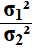or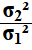. We will get a confidence interval either way, but they will be different confidence intervals. We just need to pick one of them. Right now, let us choose to find the confidence interval for.

Next we need to specify the level of confidence that we want. In this example we will look at a 90% confidence interval. That means that we want 5% of the area to the left of the confidence interval and 5% of the area to the right.

We are looking at a 90% confidence interval for. From our samples we can determine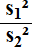, noting that this quotient corresponds to the order that we had for the population parameters,. The sample size of the numerator,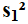, is n1. The sample size of the denominator,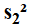, is n2. Therefore, we will use the F distribution with the degree of freedom pair (n1-1,n2-1). Within that distribution we want the x-value that has 5% of the area under the curve to the left of that value. We will call this xlow. In addition, we want the x-value that has 5% of the area under the curve to the right of that value. We will call this xhigh.

The two endpoints of our confidence interval will be (s1²/s2²)/xlow and (s1²/s2²)/xhigh. However, in doing this we get the endpoints in the opposite order. For each endpoint we are dividing s1²/s2² by a value. Dividing that quotient by a smaller value produces a larger result than dividing it by a larger value. Thus, the final confidence interval will be ( (s1²/s2²)/xhigh , (s1²/s2²)/xlow).

Case 1: Giving numbers to the general example above, we will say that we have two samples from two populations that are known to be normally distributed. The first sample has size 35 and standard deviation 4.76. The second sample has size 48 and standard deviation 5.12. Recalling that we need to look at the variances we find that the variance of the first sample is 4.76² = 22.6576 and the variance of the second sample is 5.12² = 26.2144, giving us s1²/s2² ≈ 0.8643188. We can do these computations in R with the following commands.
```s_one <- 4.76
n_one <- 35
s_two <- 5.12
n_two <- 48
v_one <- s_one^2
v_two <- s_two^2
v_one
v_two
quotient <- v_one / v_two
quotient
```
If we were in a hurry those commands would be needlessly long since we could have found that same ratio just by typing `4.76^2/5.12^2`. However, we will use the longer version so that it is easer to follow the up-coming computations. Figure 6 shows the console view of the first 8 of those commands, while Figure 6a shows the remaining two along with the one statement computation of the same quotient.
 Figure 6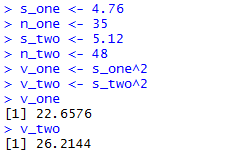Figure 6a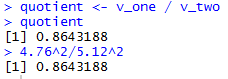Because we are dealing with s1²/s2², we know that the degrees of freedom for the F distribution will be the pair (35-1,48-1) or (34,47). To find the x-value that has 5% of the area under the curve of an F distribution, with 34,47 degrees of freedom, to left of that x-value we can use the command `qf(0.05,34,47)`. The result is 0.5808588, meaning that 5% of the area under that curve is to the left of 0.5808588. To find the x-value that has 5% of the area under the curve of an F distribution, with 34,47 degrees of freedom, to right of that x-value we can use the command `qf(0.05,34,47,lower.tail=FALSE)`. The result is 1.677293, meaning that 5% of the area under that curve is to the right of 1.677293. Slightly more elaborate versions of those commands would be
```xlow <- qf(0.05,n_one-1,n_two-1)
xhigh <- qf(0.05,n_one-1,n_two-1,lower.tail=FALSE)
xlow
xhigh
```
Figure 7 shows the R computations of those values.

Figure 7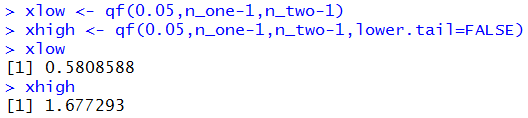To help visualize this, Figure 8 holds the plot of the F distribution for the degree of freedom pair (34,47). The x-value 0.5808588 has a blue line above it and the x-value 1.677293 has a dark green line above it. The plot was then doctored to shade the two 5% areas in yellow. (You might note that each of the little rectangles in the graph represents an area of 0.01 square units. It is worth the moment or two to convince yourself that the unshaded area under the curve amounts to 0.90 square units, the 90% we sought.)

Figure 8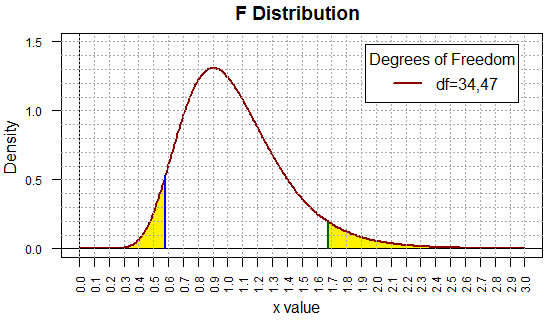Of course now that we have the xlow and xhigh values we still have the final computation to do to generate our confidence interval. That computation amounts to finding (s1²/s2²)/xhigh and (s1²/s2²)/xlow. We can do this in R via the commands
```c_low <- quotient/xhigh
c_high <- quotient/xlow
c_low
c_high
```
remembering that to get the low end of the confidence interval we divide that quotient by the larger value, xhigh, whereas to get the upper end of the confidence interval we divide the quotient by the smaller value, xlow. Figure 9 shows the result of those commands.

Figure 9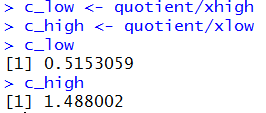Thus, our 90% confidence interval for the ratio of the population variances,, is (0.515,1.488).

Case 1 (revisited): Rather than just jumping into a completely new example, we will redo the previous case, but this time we look at the inverse ratio. That is, we will look for the 90% confidence interval for the ratio of.

I can see three ways to approach this new problem. The first is to use the values assigned above in Figure 6. Then we could redo the commands shown in Figures 6a, swapping the placement of v_one and v_two, as well as the commands in Figure 7 swapping the placement of n_one and n_two. The computation in Figure 9 stays the same. Thus the sequence of commands would be
```# now do it over, swapping variables
s_one <- 4.76
n_one <- 35
s_two <- 5.12
n_two <- 48
v_one <- s_one^2
v_two <- s_two^2
v_one
v_two
quotient <- v_two / v_one
xlow <- qf(0.05,n_two-1,n_one-1)
xhigh <- qf(0.05,n_two-1,n_one-1,lower.tail=FALSE)
xlow
xhigh
c_low <- quotient/xhigh
c_high <- quotient/xlow
c_low
c_high
```
The console view of those commands is given in Figure 10 and Figure 10a.
 Figure 10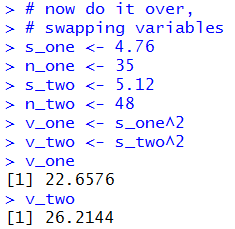Figure 10a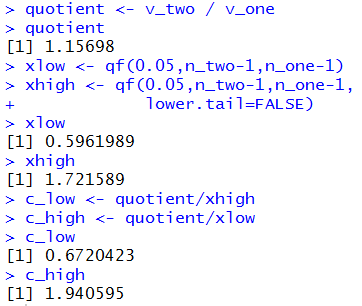As you can see we have a new ratio, 1.15698, new low and high F values, 0.5962989 and 1.721589 (because the order of the degree of freedom values has changed), and a new confidence interval (0.672,1.94).

A second approach to solving this new case would have been to just swap the original values. Yes, we want to find the confidence interval for, and yes the original statements found the confidence interval for, but the original associated n_one and s_one with the values for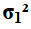. Why not leave the commands as they were and just associate n_one and s_one with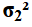, and associate n_two and s_two with? Doing that would produce the sequence of commands
```# now do it over,
# but reverse the samples
s_one <- 5.12
n_one <- 48
s_two <- 4.76
n_two <- 35
v_one <- s_one^2
v_two <- s_two^2
v_one
v_two
quotient <- v_one / v_two
quotient
xlow <- qf(0.05,n_one-1,n_two-1)
xhigh <- qf(0.05,n_one-1,n_two-1,
lower.tail=FALSE)
xlow
xhigh
c_low <- quotient/xhigh
c_high <- quotient/xlow
c_low
c_high
```

 Figure 11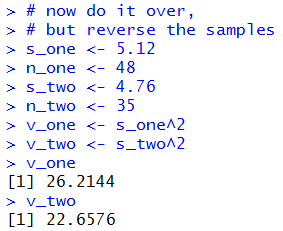Figure 11a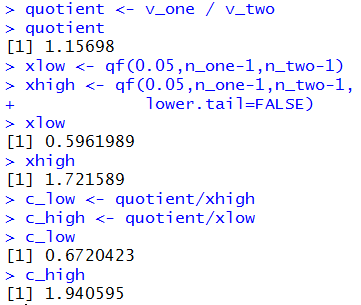Of course, we get the same results in Figure 11a as we did in Figure 10a.

The first approach had us changing the real computational commands while the second had us changing the association of values to variables. Both are somewhat confusing. Here is a third approach. Change the commands, once and for all, to use variables that are just associated with the top or bottom variance rather than being associated with a numbered, 1 or 2, variance. Now the commands appear as
```# now do it over,
# but reverse use new,
# more general, variables
s_top <- 5.12
n_top <- 48
s_bot <- 4.76
n_bot <- 35
v_top <- s_top^2
v_bot <- s_bot^2
v_top
v_bot
quotient <- v_top / v_bot
quotient
xlow <- qf(0.05,n_top-1,n_bot-1)
xhigh <- qf(0.05,n_top-1,n_bot-1,
lower.tail=FALSE)
xlow
xhigh
c_low <- quotient/xhigh
c_high <- quotient/xlow
c_low
c_high
```
Figure 12 and 12a show the console view of those commands.
 Figure 12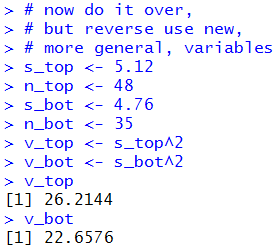Figure 12a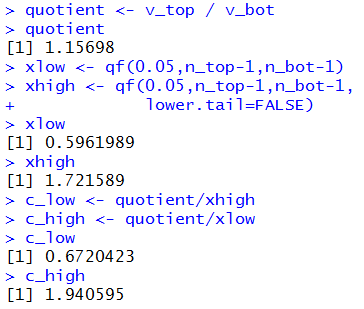Before we leave this case, we should at least look at the appropriate F distribution, noting the cutoff points that we found in Figure 12a, namely, 0.5961989 and 1.721589. The plot of that F distribution is given in Figure 13. As in Figure 8, this image has been doctored to show the two 5% regions in yellow.

Figure 13The beauty of this new set of commands, given above Figure 12, is that we can apply it, logically, to any new problem. For example, if we have two normal populations, one of Ford products and one of General Motors products, and we want to get a 90% confidence interval for the quotient of the variance of the GM products to the variance of the Ford products, then we can take two random samples and get their sample size and sample standard deviation. Then, we plug in the GM values for the top variables and the Ford sample values for the bot variables and all of the rest of the computations stay the same.

Case 2: We have such a case. The Ford sample is of size 67 with standard deviation of 14.27 while the GM sample is of size 13 with sample standard deviation of 11.34. We know that the GM values are to be associated with the top variables. Thus, our commands become
```# Case 2
s_top <- 11.34
n_top <- 13
s_bot <- 14.27
n_bot <- 67
v_top <- s_top^2
v_bot <- s_bot^2
v_top
v_bot
quotient <- v_top / v_bot
quotient
xlow <- qf(0.05,n_top-1,n_bot-1)
xhigh <- qf(0.05,n_top-1,n_bot-1,
lower.tail=FALSE)
xlow
xhigh
c_low <- quotient/xhigh
c_high <- quotient/xlow
c_low
c_high
```
They produce the console views shown in Figure 14 and Figure 14a.
 Figure 14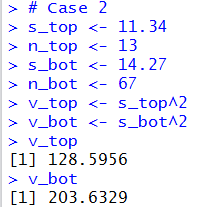Figure 14a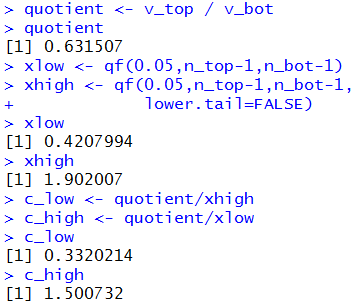Therefore, our 90% confidence interval for the ratio of the GM variance to the Ford variance is (0.332,1.501). While we are at it, Figure 15 shows the marked-up F distribution that we would be using for this problem.

Figure 15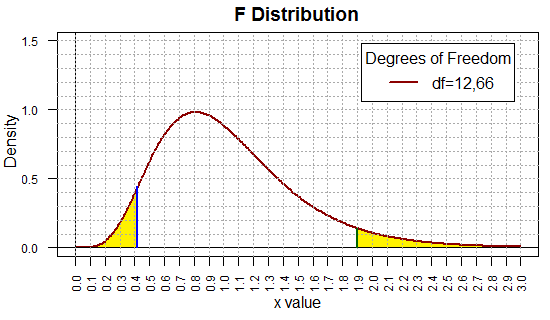Although it is nice to have this general set of commands to do our work, it would be even nicer to capture them in a function. When we do this we will want to add a specification for the confidence level. Consider the following function definition:
```ci_2popvar <- function (
n_top, s_top, n_bot, s_bot,
cl=0.95)
{ # generate a confidence interval for the  ratio
# of the top variance to the bottom variance from
# a sample of the top population with size n_top
# and standard deviation s_top and a sample of the
# bottom population with size n_bot and standard
# deviation s_bot.  cl gives the confidence level.

alpha <- 1-cl
v_top <- s_top^2
v_bot <- s_bot^2
quotient <- v_top / v_bot
lower.tail=FALSE)
c_low <- quotient/xhigh
c_high <- quotient/xlow

result <- c( c_low, c_high, quotient,
xlow, xhigh, v_top,
s_top, n_top, v_bot, s_bot,
names(result) <-
c( "CI Low", "CI_HIGH", "Quotient",
"F Low",  "F High",  "Top Var.",
"Top sd", "Top size", "Bot Var.",
"Bot sd", "Bot size", "C level",
"Alpha/2")
return( result)
}
```
With that function placed in the parent directory we can use the commands
```source("../ci_2popvar.R")
ci_2popvar(48, 5.12, 35, 4.76, 0.90)
ci_2popvar(13, 11.34, 67, 14.27, 0.90)
```
to do all of the work in Figures 12, 12a, 14, and 14a. The results are shown in Figure 16.

Figure 16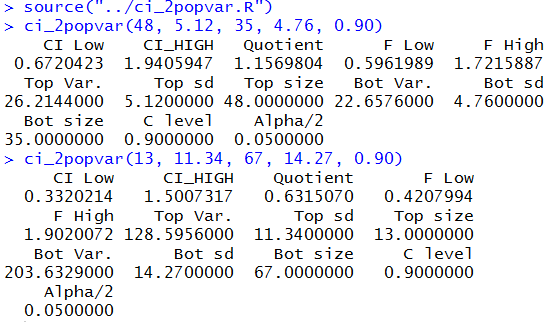Then, too, with a simple change we can do that same work again, but this time to get a 98% confidence interval. Those commands would be
```ci_2popvar(48, 5.12, 35, 4.76, 0.98)
ci_2popvar(13, 11.34, 67, 14.27, 0.98)
```
and their results are shown in Figure 17.

Figure 17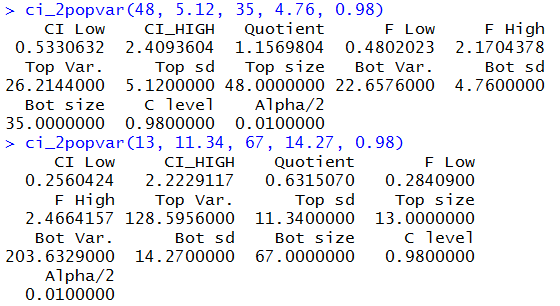### Hypothesis test

Having worked our way through the construction of confidence intervals our next challenge is to construct a test for the null hypothesis that two populations, known to be normal, have the same variance. That is, we want to have a test for H0: σ1² = σ2². As we have seen before, we will have to have an alternative hypothesis and it will take the form of one of
• H1: σ1² ≠ σ2².
• H1: σ1² < σ2².
• H1: σ1² > σ2².
To do this test we will take two samples, one from each population, and we will find the variance of each sample. If the null hypothesis is true then we expect that the two sample variances will be approximately equal. Evidence to indicate that the null hypothesis is not true would be to find that the sample variance differs significantly.

In earlier hypothesis tests we looked for such evidence by finding the difference of the sample statistics. We did that because we knew, in those earlier situations, the distribution of the difference of the sample statistics. Here we know the distribution of the ratio of the sample statistics, that is, we know that s1² / s2² is a F distribution with the degree of freedom pair (n1-1, n2-1).

Using this we can restate the null hypothesis as H0: σ1² / σ2² = 1. and the alternative hypotheses as
• H1: σ1² / σ2² ≠ 1.
• H1: σ1² / σ2² < 1.
• H1: σ1² / σ2² > 1.
To reject H0 in favor of H1: σ1² / σ2² ≠ 1. we will need to have the value of s1² / s2² be far enough away, in either direction, from 1 that the probability of getting that kind of value will be less than the stated level of significance. To reject H0 in favor of H1: σ1² / σ2² < 1. we will need to have the value of s1² / s2² be far enough away, toward 0, from 1, that the probability of getting that kind of value will be less than the stated level of significance. To reject H0 in favor of H1: σ1² / σ2² > 1. we will need to have the value of s1² / s2² be far enough away, above 1, from 1, that the probability of getting that kind of value will be less than the stated level of significance. Let us try a few examples.

Case 3: We have two populations, both known to be normal and we want to test H0: σ1² / σ2² = 1. against the alternative hypothesis H1: σ1² / σ2² ≠ 1. at the 0.05 level of significance. We take samples from the two populations. The sample from the first population is of size 36 and it has a sample standard deviation of 23.14, therefore the sample variance is 23.14² or 535.4596. The sample from the second population is of size 58 and it has a sample standard deviation of 28.31, therefore the sample variance is 28.31² or 801.4561. The quotient of the variances will be 535.4596 / 801.4561 which is about 0.6681.

The ratio of the variances will have a F distribution with the degree of freedom pair (35,57). Since this is a 2-sided test, for that degree of freedom pair we want the x-value that has 0.025 of the area to its left and the x-value that has 0.025 of the area to its right. Those values are 0.535 and 1.7888, respectively. These are our critical values. But our quotient was 0.6681 and this is not outside of our critical values so we say that we do not have sufficient evidence to reject H0.

For the attained significance approach, we need to find the probability of getting a ratio more extreme than 0.6681. This ratio is less than 1 so we want to find the area under the F-distribution with 35 and 57 degrees of freedom to the left of 0.6681. Once we get that value, we need to double it to account for upper tail. The left-tail value is about 0.10176. Therefore, our attained significance would be 0.20352 a value that is not less than the specified level of significance, 0.05, so we say that we do not have sufficient evidence to reject H0.

The R statements to do these calculations could be:
```# hypothesis testing
n_top <- 36
s_top <- 23.14
n_bot <- 58
s_bot <- 28.31
v_top <- s_top^2
v_bot <- s_bot^2
v_top
v_bot
quotient <- v_top / v_bot
quotient
xlow <- qf(0.025,n_top-1,n_bot-1)
xhigh <- qf(0.025,n_top-1,n_bot-1,
lower.tail=FALSE)
# critical values
xlow
xhigh
# attained significance
pf(quotient,n_top-1,n_bot-1)
pf(quotient,n_top-1,n_bot-1)*2
```
The console view of those statements is shown in Figure 18 and Figure 18a.
 Figure 18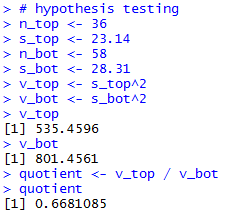Figure 18a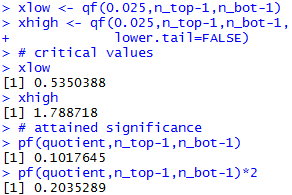Case 3 (reversed): For this case we use exactly the same values as in the previous case but we will reverse the populations. That is, instead of looking at the ratio H0: σ1² / σ2² = 1 we will look at the ratio H0: σ2² / σ1² = 1. The alternative hypothesis is now H1: σ2² / σ1² ≠ 1.

We have the same samples, so they have the same variances. However, this time we will look at the sample statistic s2² / s1². Again, in order to reject H0 the particular value of that sample statistic will have to be sufficiently far from 1. The distribution of such a sample statistic is a F distribution with (58-1,36-1) degrees of freedom. It is important to note that we had to reverse the order of those values because the top (numerator) of the ratio s2² / s1² is the s2 and the first of the pair of the degrees of freedom is associated with the top (numerator) value in the quotient. Of course, that change means that we have to again find the x-value in the F distribution with (57,35) degrees of freedom that has 2.5% of the area to its left and we need to find the similar value that has 2.5% of the area to its right. These values, which are different from the ones we found above because the degrees of freedom changed, are 0.559 and 1.869, respectively. These are the critical values. When we calculate the ratio 801.4561 / 535.4596 we get 1.497, and this is not extreme enough to reject the null hypothesis in favor of the alternative at the 0.05 level of significance.

We could have used the attained significance approach and looked at how "strange" is it to get the ratio 1.497 if the null hypothesis is true. Since that ratio is greater than 1 we need to look at the probability of getting that value or higher if H0 is true. That probability turns out to be 0.10176. Of course, since this is a two-tail test we need to double that to account for being "that far" off in the other direction. Therefore, the attained significance is, again, 0.20352, a value that is not less than the level of significance specified, 0.05, so we do not have sufficient evidence to reject the null hypothesis in favor of the alternative hypothesis. You might have noticed that this attained significance is exactly the same as the attained significance in the original Case 3 example. There, with (35,57) degrees of freedom we looked at the left tail and then doubled it, here with (57,35) degrees of freedom, we looked at the right tail and then doubled it. With those correct interpretations we certainly should get the same attained significance for the samples that we have.

Other than changing the computation of the attained significance to use the upper tail, and of course changing the initial assignments of values, the R statements remain the same.
```n_top <- 58
s_top <- 28.31
n_bot <- 36
s_bot <- 23.14
v_top <- s_top^2
v_bot <- s_bot^2
v_top
v_bot
quotient <- v_top / v_bot
quotient

xlow <- qf(0.025,n_top-1,n_bot-1)
xhigh <- qf(0.025,n_top-1,n_bot-1,
lower.tail=FALSE)
# critical values
xlow
xhigh
# attained significance
pf(quotient,n_top-1,n_bot-1,
lower.tail=FALSE)
pf(quotient,n_top-1,n_bot-1,
lower.tail=FALSE)*2
```
The console view of those statements is given in Figure 19 and Figure 19a.
 Figure 19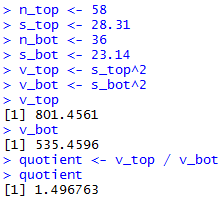Figure 19a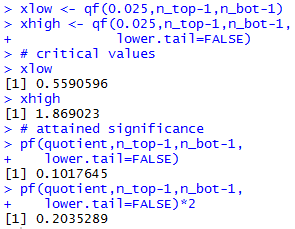Case 4:We have two normally distributed populations. We want to test the hypothesis that they have equal variances against the alternative that the variance of the first is greater than the variance of the second. We note that if the first is greater than the second then the first divided by the second will be greater than 1. We want to run this test at the 0.01 level of significance.

We take two samples and find that the sample from the first population is of size 87 and it has a sample standard deviation of 32.19 while the sample from the second population is of size 43 and it has a standard deviation of 48.52. From this information we see that the first sample variance is 1036.196 and the second sample variance is 2354.19, giving us the ratio 0.44. We can stop our analysis right there. The alternative is that the first variance is greater than the second, equivalently that the ratio of the first to the second is greater than 1. To reject the null hypothesis, we need to have the samples give us a ratio that is significantly larger than 1. The samples that we have yield a ratio that is less than 1. This is certainly no evidence that we should reject H0 in favor of H1.

Of course we could find the critical value. We are looking for the x-value for the F distribution curve with the degree of freedom pair (86,42) that has 1% of the area to its right. That turns out to be about 1.9317. We would have enough evidence to reject H0 if the sample statistic is greater than the critical value. Clearly, 0.44 is not greater than 1.9317. We do not have evidence to reject H0 in favor of the alternative.

The attained significance of our absurdly low ratio is 0.999 which is clearly not less than the 0.01 significance level of the test.

The R commands that produced those values follow.
```
# case 4
n_top <- 87
s_top <- 32.19
n_bot <- 43
s_bot <- 48.52
v_top <- s_top^2
v_bot <- s_bot^2
v_top
v_bot
quotient <- v_top / v_bot
quotient

xhigh <- qf(0.01,n_top-1,n_bot-1,
lower.tail=FALSE)
# critical value
xhigh
# attained significance
pf(quotient,n_top-1,n_bot-1,
lower.tail=FALSE)
```
Case 5: For this example we will repeat the previous one but with a different alternative hypothesis. Now H1: σ1² / σ2² < 1. With this as the alternative we need to have the ratio of the sample variances be far below 1 in order to reject the null hypothesis. How far below 1 do we need to be? The x-value in the F distribution with the degrees of freedom pair (86,42) that has 1% of the area to its left is 0.5504748. Thus, our one critical value for this one-tail test is 0.5504748. The ratio of the variances is 1036.196 / 2354.19 ≈ 0.44, a value that is even further away from 1 than is the critical value. Therefore, our samples give us sufficient evidence to reject H0 in favor of H1 at the 0.01 level of significance.

The R statements for those computations follow.
```n_top <- 87
s_top <- 32.19
n_bot <- 43
s_bot <- 48.52
v_top <- s_top^2
v_bot <- s_bot^2
v_top
v_bot
quotient <- v_top / v_bot
quotient

xlow <- qf(0.01,n_top-1,n_bot-1)

# critical value
xlow
# attained significance
pf(quotient,n_top-1,n_bot-1)
```
The console view of the statements is given in Figure 20 and Figure 20a.
 Figure 20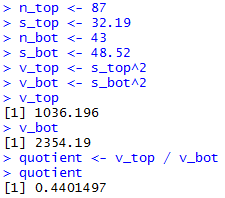Figure 20a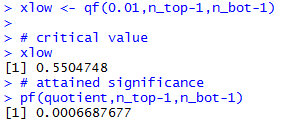We have seen that pretty much the same statements are used to solve all of our problems. We want to capture these in a function. Here is one such function.
```hypoth_2test_var <- function(
n_top, s_top, n_bot, s_bot,
H1_type, sig_level=0.05)
{ # perform a hypothsis test for equality of
# the variances of two populations
# based on two samples of n_top and n_not
# items yielding sample
# standard deviations s_top and s_bot
#  where the alternative hypothesis  is
#      !=  if H1_type==0
#      <   if H1_type < 0
#      >   if H1_type > 0
# Do the test at sig_level significance,
#
# It is important that the population is a normal
# distribution.

decision <- "do not reject"
v_top = s_top^2
v_bot = s_bot^2
quotient <- v_top / v_bot
alpha <- sig_level
if ( H1_type == 0 )
{ alpha <- alpha/2 }
#find the critical value(s)
xlow <- qf(alpha,n_top-1,n_bot-1)
xhigh <- qf( alpha, n_top-1, n_bot-1,
lower.tail=FALSE)
if( H1_type == 0 )
{  if( (quotient < xlow) | (quotient>xhigh) )
{ decision <- "Reject" }
if (quotient < 1 )
{ attained = 2*pf(quotient,n_top-1,n_bot-1)}
else
{ attained = 2*pf(quotient, n_top-1,
n_bot-1, lower.tail=FALSE) }
H1 <- "v_1 != v_2"
}
else if( H1_type < 0 )
{  if( quotient < xlow )
{ decision <- "Reject" }
attained = pf( quotient,n_top-1,n_bot-1)
xhigh <- "n.a."
H1 <- "v_1 < v_2"
}
else
{  if( quotient > xhigh )
{ decision <- "Reject" }
attained = pf( quotient,n_top-1,
n_bot-1,lower.tail=FALSE)
xlow <- "n.a."
H1 <- "v_1 > v_2"
}
result <- c( H1, n_top, s_top, v_top,
n_bot, s_bot, v_bot, quotient, xlow,
xhigh, decision, attained )
names(result) <- c( "H1","n top", "s top", "v top",
"n bot", "s bot", "v bot",
"quotient", "crit low", "crit high",
"decision", "attained")
return (result)
}
```
Then, assuming we have placed the source code for that function in a file named hypo_2var.R in the parent directory (folder), we could load the function and do all four of the tests above via the statements
```source("../hypo_2var.R")
#case 3
hypoth_2test_var( 36, 23.14, 58, 28.31, 0, 0.05)
#case 3 reversed
hypoth_2test_var( 58, 28.31, 36, 23.14, 0, 0.05)
#case 4
hypoth_2test_var(87, 32.19, 43, 48.52, 1, 0.01)
#case 5
hypoth_2test_var(87, 32.19, 43, 48.52, -1, 0.01)
```
The console view of those is given in Figures 21 through 24.

Figure 21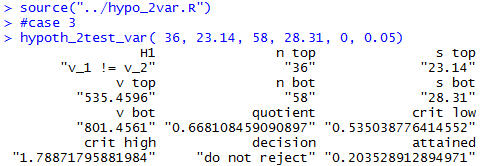Figure 22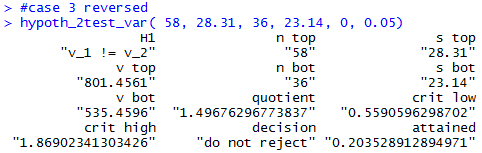Figure 23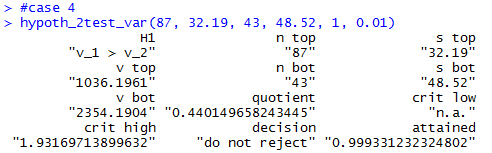Figure 24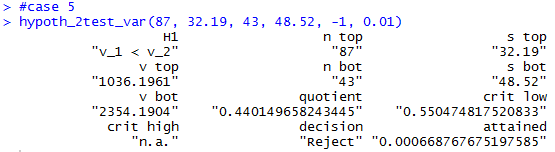Below is a listing of the R commands used in generating plots in Figures 1 through 5 above.
```# Display the F distributions with
# four different sets of pairs of degrees of freedom.

x <- seq(0, 4.5, length=200)
hx <- rep(0,200)

degf1 <- c(60,60,10,8)
degf2 <- c(60,10,60,40)
colors <-  c("red",  "black", "darkgreen", "blue")
labels <- c("df=60,60",  "df=60,10",  "df=10,60",  "df=8,40")

plot(x, hx, type="n", lty=2, lwd=2, xlab="x value",
ylab="Density", ylim=c(0,1.7), xlim=c(0,4), las=1,
xaxp=c(0,4,8),
main="F Distribution "
)

for (i in 1:4){
lines(x, df(x,degf1[i],degf2[i]), lwd=2, col=colors[i], lty=1)
}
abline(h=0)
abline(h=seq(0.1,1.7,0.1), lty=3, col="darkgray")
abline(v=0)
abline(v=seq(0.5,4,0.5), lty=3, col="darkgray")
legend("topright", inset=.05, title="Degrees of Freedom",
labels, lwd=2, lty=1, col=colors)

for (j in 1:4 ){
plot(x, hx, type="n", lty=2, lwd=2, xlab="x value",
ylab="Density", ylim=c(0,1.7), xlim=c(0,4), las=1,
xaxp=c(0,4,8),
main=paste("F Distribution: Degrees of Freedom ",labels[j])
)

for (i in j:j){
lines(x, df(x,degf1[i],degf2[i]), lwd=2, col=colors[i], lty=1)
}
abline(h=0)
abline(h=seq(0.1,1.7,0.1), lty=3, col="darkgray")
abline(v=0)
abline(v=seq(0.5,4,0.5), lty=3, col="darkgray")
legend("topright", inset=.05, title="Degrees of Freedom",
labels[j], lwd=2, lty=1, col=colors[j])

}
```

Here is a listing of the computational commands used in creating this page:
```s_one <- 4.76
n_one <- 35
s_two <- 5.12
n_two <- 48
v_one <- s_one^2
v_two <- s_two^2
v_one
v_two
quotient <- v_one / v_two
quotient
4.76^2/5.12^2

xlow <- qf(0.05,n_one-1,n_two-1)
xhigh <- qf(0.05,n_one-1,n_two-1,lower.tail=FALSE)
xlow
xhigh

x <- seq(0, 3, length=300)
hx <- rep(0,300)
degf1 <- n_one - 1
degf2 <- n_two - 1
colors <- "darkred"
labels <- paste("df=",n_one-1,",",n_two-1,sep="")
plot(x, hx, type="n", lty=2, lwd=2, xlab="x value",
ylab="Density", ylim=c(0,1.5), xlim=c(0,3), las=2,
xaxp=c(0,3,30),cex.axis=0.75,
main="F Distribution "
)
lines(x, df(x,degf1,degf2), lwd=2, col=colors, lty=1)
abline(h=0)
abline(h=seq(0.1,1.5,0.1), lty=3, col="darkgray")
abline(v=0)
abline(v=seq(0.0,3,0.1), lty=3, col="darkgray")
legend("topright", inset=.05, title="Degrees of Freedom",
labels, lwd=2, lty=1, col=colors)
lines(c(xlow,xlow),c(0,df(xlow,degf1,degf2)),col="blue",lw=2)
lines(c(xhigh,xhigh),c(0,df(xhigh,degf1,degf2)),col="darkgreen",lw=2)

c_low <- quotient/xhigh
c_high <- quotient/xlow
c_low
c_high

# now do it over,
# swapping variables
s_one <- 4.76
n_one <- 35
s_two <- 5.12
n_two <- 48
v_one <- s_one^2
v_two <- s_two^2
v_one
v_two
quotient <- v_two / v_one
quotient
xlow <- qf(0.05,n_two-1,n_one-1)
xhigh <- qf(0.05,n_two-1,n_one-1,
lower.tail=FALSE)
xlow
xhigh
c_low <- quotient/xhigh
c_high <- quotient/xlow
c_low
c_high

# now do it over,
# but reverse the samples
s_one <- 5.12
n_one <- 48
s_two <- 4.76
n_two <- 35
v_one <- s_one^2
v_two <- s_two^2
v_one
v_two
quotient <- v_one / v_two
quotient
xlow <- qf(0.05,n_one-1,n_two-1)
xhigh <- qf(0.05,n_one-1,n_two-1,
lower.tail=FALSE)
xlow
xhigh
c_low <- quotient/xhigh
c_high <- quotient/xlow
c_low
c_high

# now do it over,
# but reverse use new,
# more general, variables
s_top <- 5.12
n_top <- 48
s_bot <- 4.76
n_bot <- 35
v_top <- s_top^2
v_bot <- s_bot^2
v_top
v_bot
quotient <- v_top / v_bot
quotient
xlow <- qf(0.05,n_top-1,n_bot-1)
xhigh <- qf(0.05,n_top-1,n_bot-1,
lower.tail=FALSE)
xlow
xhigh
c_low <- quotient/xhigh
c_high <- quotient/xlow
c_low
c_high

x <- seq(0, 3, length=300)
hx <- rep(0,300)
degf_top <- n_top - 1
degf_bot <- n_bot - 1
colors <- "darkred"
labels <- paste("df=",n_top-1,",",n_bot-1,sep="")
plot(x, hx, type="n", lty=2, lwd=2, xlab="x value",
ylab="Density", ylim=c(0,1.5), xlim=c(0,3), las=2,
xaxp=c(0,3,30),cex.axis=0.75,
main="F Distribution "
)
lines(x, df(x,degf_top,degf_bot), lwd=2, col=colors, lty=1)
abline(h=0)
abline(h=seq(0.1,1.5,0.1), lty=3, col="darkgray")
abline(v=0)
abline(v=seq(0.0,3,0.1), lty=3, col="darkgray")
legend("topright", inset=.05, title="Degrees of Freedom",
labels, lwd=2, lty=1, col=colors)
lines(c(xlow,xlow),c(0,df(xlow,degf_top,degf_bot)),col="blue",lw=2)
lines(c(xhigh,xhigh),c(0,df(xhigh,degf_top,degf_bot)),col="darkgreen",lw=2)

# Case 2
s_top <- 11.34
n_top <- 13
s_bot <- 14.27
n_bot <- 67
v_top <- s_top^2
v_bot <- s_bot^2
v_top
v_bot
quotient <- v_top / v_bot
quotient
xlow <- qf(0.05,n_top-1,n_bot-1)
xhigh <- qf(0.05,n_top-1,n_bot-1,
lower.tail=FALSE)
xlow
xhigh
c_low <- quotient/xhigh
c_high <- quotient/xlow
c_low
c_high

x <- seq(0, 3, length=300)
hx <- rep(0,300)
degf_top <- n_top - 1
degf_bot <- n_bot - 1
colors <- "darkred"
labels <- paste("df=",n_top-1,",",n_bot-1,sep="")
plot(x, hx, type="n", lty=2, lwd=2, xlab="x value",
ylab="Density", ylim=c(0,1.5), xlim=c(0,3), las=2,
xaxp=c(0,3,30),cex.axis=0.75,
main="F Distribution "
)
lines(x, df(x,degf_top,degf_bot), lwd=2, col=colors, lty=1)
abline(h=0)
abline(h=seq(0.1,1.5,0.1), lty=3, col="darkgray")
abline(v=0)
abline(v=seq(0.0,3,0.1), lty=3, col="darkgray")
legend("topright", inset=.05, title="Degrees of Freedom",
labels, lwd=2, lty=1, col=colors)
lines(c(xlow,xlow),c(0,df(xlow,degf_top,degf_bot)),col="blue",lw=2)
lines(c(xhigh,xhigh),c(0,df(xhigh,degf_top,degf_bot)),col="darkgreen",lw=2)

source("../ci_2popvar.R")
ci_2popvar(48, 5.12, 35, 4.76, 0.90)
ci_2popvar(13, 11.34, 67, 14.27, 0.90)
#
ci_2popvar(48, 5.12, 35, 4.76, 0.98)
ci_2popvar(13, 11.34, 67, 14.27, 0.98)
#

# hypothesis testing
n_top <- 36
s_top <- 23.14
n_bot <- 58
s_bot <- 28.31
v_top <- s_top^2
v_bot <- s_bot^2
v_top
v_bot
quotient <- v_top / v_bot
quotient
xlow <- qf(0.025,n_top-1,n_bot-1)
xhigh <- qf(0.025,n_top-1,n_bot-1,
lower.tail=FALSE)
# critical values
xlow
xhigh
# attained significance
pf(quotient,n_top-1,n_bot-1)
pf(quotient,n_top-1,n_bot-1)*2

# redo the example but reverse the
# ratio, so degrees of freedom
# reverse
n_top <- 58
s_top <- 28.31
n_bot <- 36
s_bot <- 23.14
v_top <- s_top^2
v_bot <- s_bot^2
v_top
v_bot
quotient <- v_top / v_bot
quotient

xlow <- qf(0.025,n_top-1,n_bot-1)
xhigh <- qf(0.025,n_top-1,n_bot-1,
lower.tail=FALSE)
# critical values
xlow
xhigh
# attained significance
pf(quotient,n_top-1,n_bot-1,
lower.tail=FALSE)
pf(quotient,n_top-1,n_bot-1,
lower.tail=FALSE)*2

# case 4
n_top <- 87
s_top <- 32.19
n_bot <- 43
s_bot <- 48.52
v_top <- s_top^2
v_bot <- s_bot^2
v_top
v_bot
quotient <- v_top / v_bot
quotient

xhigh <- qf(0.01,n_top-1,n_bot-1,
lower.tail=FALSE)
# critical value
xhigh
# attained significance
pf(quotient,n_top-1,n_bot-1,
lower.tail=FALSE)

# case 5
n_top <- 87
s_top <- 32.19
n_bot <- 43
s_bot <- 48.52
v_top <- s_top^2
v_bot <- s_bot^2
v_top
v_bot
quotient <- v_top / v_bot
quotient

xlow <- qf(0.01,n_top-1,n_bot-1)

# critical value
xlow
# attained significance
pf(quotient,n_top-1,n_bot-1)

# define our function

source("../hypo_2var.R")
#case 3
hypoth_2test_var( 36, 23.14, 58, 28.31, 0, 0.05)
#case 3 reversed
hypoth_2test_var( 58, 28.31, 36, 23.14, 0, 0.05)
#case 4
hypoth_2test_var(87, 32.19, 43, 48.52, 1, 0.01)
#case 5
hypoth_2test_var(87, 32.19, 43, 48.52, -1, 0.01)
```